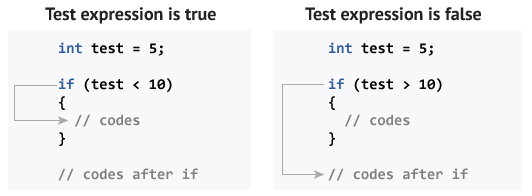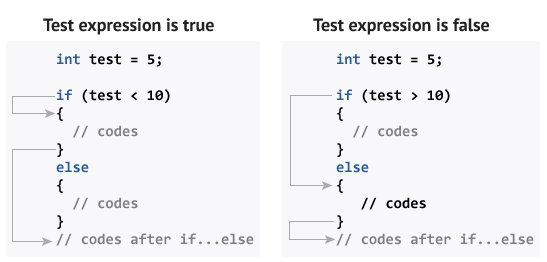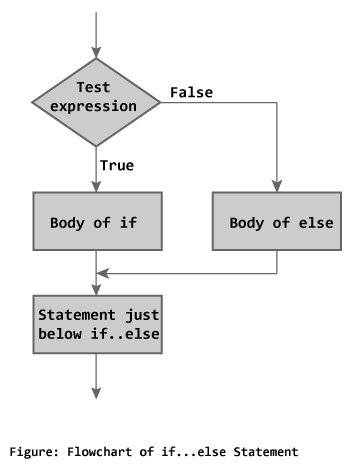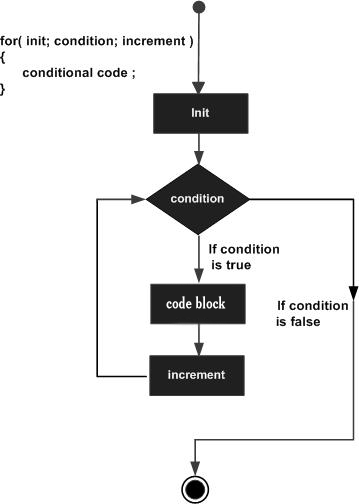### Object Oriented Programming

#### Unit 2 :-Control Structure

Decision making Statement
if -else:-

The `if` statement evaluates the test expression inside parenthesis.
If test expression is evaluated to true, Then statements inside the body of `if` is executed.
If test expression is evaluated to false, Then statements inside the body of `if` is skipped(not exicute).// Program to print Even number entered by the user

```// If user enters Even number, it is skipped #include <iostream.h> int main() { int number; cout << "Enter an integer: "; cin >> number; // checks if the number is positive if ( number%2==0) { cout << "You entered a Even integer: " << number << endl; } ```

```getch(); return 0;} ```

else if// Program to print Even number Or Not entered by the user

```// If user enters Even number,then print number is even else odd #include <iostream.h> int main() { clrscr(); int number; cout << "Enter an integer: "; cin >> number; // checks if the number is positive if ( number%2==0) { cout << "You entered a Even integer: " << number << endl; } else{cout<<"````You entered a Odd integer: " << number << endl;``} `

``````return 0;
getch(); }``````

## C++ Nested if…else

The `if...else` statement executes two different codes depending upon whether the test expression is true or false. Sometimes, a choice has to be made from more than 2 possibilities.

The nested `if...else` statement allows you to check for multiple test expressions and execute different codes for more than two conditions.

### Syntax of Nested if…else

if (testExpression1)
{ // statements to be executed if testExpression1 is true }
else if(testExpression2)
{ // statements to be executed if testExpression1 is false and testExpression2 is true }
else if (testExpression 3)
{ // statements to be executed if testExpression1 and testExpression2 is false and testExpression3 is true }
. .
else { // statements to be executed if all test expressions are false }

### Example 3: C++ Nested if…else

```// Program to check whether an integer is positive, negative or zero #include <iostream.h> int main() { clrscr(); int number; cout << "Enter an integer: "; cin >> number; if ( number > 0) { cout << "You entered a positive integer: " << number << endl; } else if (number < 0) { cout<<"You entered a negative integer: " << number << endl; } else { cout << "You entered 0." << endl; } cout << "This line is always printed."; return 0;```

``````getch();
}``````

Output

Enter an integer: 0
You entered 0.
This line is always printed.

## Conditional/Ternary Operator ?:

A ternary operator operates on 3 operands which can be used instead of a `if...else`statement.

Consider this code:

if ( a < b ) { a = b; }
else { a = -b; }

You can replace the above code with:

```a = (a < b) ? b : -b;
```

The ternary operator is more readable than a `if...else` statement for short conditions.

Switch case

switch case is used in basicly Menu drive programing#include<iostream.h>
#include<conio.h>
#include<process.h>//for exit(0);
int main()
cout<<“2:-Subtraction”;
cout<<“3:-Multiple”;
cout<<“4:-EXIT”;
cin<<ch;
switch(ch)
{ case 1:  cout<<“enter two no:-“;
break;//for out the case
case 2:  cout<<“enter two no:-“;
cin>>a>>b; sub=a-b;
cout<<“subtraction=”<<sub; break;
case 3:
cout<<“enter two no:-“;
cin>>a>>b; mul=a*b;
cout<<“Multiplication=”<<mul;
break;
case 4:   exit(0);
break;   } // end of switch
getch(); }

FOR LOOP

Syntax:-   for(initialize;condition;increment/decremenr)
Flow ChartExample:-write a program to print table?

```#include<iostream.h>
#include<conio.h>
int main()
{
int no,table,i;
cout<<"enter a number";
cin>>no;
for(i=1;i<=10;i++)
{
table=no*i;
cout<<no<<"*"<<i<<"="<<table;
}
getch();
}

output:-
enter a number:-5
5*1=5
5*2=10
5*3=15
.....
.....```

NEXT Unit Is coming soon………….

1.•2.